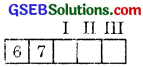# GSEB Solutions Class 11 Maths Chapter 7 Permutations and Combinations Ex 7.1

Gujarat Board GSEB Textbook Solutions Class 11 Maths Chapter 7 Permutations and Combinations Ex 7.1 Textbook Questions and Answers.

## Gujarat Board Textbook Solutions Class 11 Maths Chapter 7 Permutations and Combinations Ex 7.1

Question 1.
How many 3-digit numbers can be formed from the digits 1, 2, 3, 4 and 5, assuming.
(i) repetition of digits is allowed?
(ii) repetition of digits is not allowed?
Solution:
(i) There are five digits, viz. 1, 2, 3, 4 and 5.
Every digit can be selected any number of times.
Hence, we can select first digit 5 times.
The second digit 5 times and the third digit 5 times.
Hence, the number of ways in which the selection of three digits can be made = 5 × 5 × 5 ways = 125 ways.
(ii) Under the restriction, first digit can be selected in 5 ways. After the selection of first digit, four digits are left. Second digit can be selected in 4 ways and third digit can be selected in 3 ways.
The total number of ways of selecting three digits is 5 × 4 × 3 = 60.Question 2.
How many 3-digit even numbers can be formed from the digits 1, 2, 3, 4, 5, 6, if the digits can be repeated?
Solution:
Let 2 be fixed at unit’s place. The ten’s place can be filled up in 6 ways. The hundred’s place can also be filled in 6 ways.
Number of numbers that can be formed when 2 is at unit’s place = 6 × 6 = 36.
Similarly, when 4 is at unit’s place, the number of numbers that can be formed = 36.
Again, when 6 is at the unit’s place, the number of numbers that can be formed = 36.
∴ The total numbers of ways when 3-digits numbers can be formed, the digits being repeated = 36 × 3 = 108.Question 3.
How many 4-letter code words are possible, using the first 10 letters of the English alphabet, if no letter can be repeated?
Solution:
First letter of the code word can be selected in 10 ways. After the selection of the first letter, we have 9 letters. Hence, the second letter can be selected in 9 ways. According to FPC, first two letters can be selected in 10 × 9 ways.
Similarly, third letter can be selected in 8 way s and the fourth letter can be selected in 7 ways. According to FPC, first two letter can be selected in 7 ways. According to FPC, number of ways of selecting four letters out of 10 letters of English alphabet
= 10 × 9 × 8 × 7 ways
= 5040 ways.Question 4.
How many 5 – digit telephone numbers can be constructed using the digits 0 to 9, if each number starts with 67 and no digit appears more than once?
Solution:
There are 10 digits from 0 to 9.
I place after 67 can be filled in 8 ways.
II place after 67 can be filled in 7 ways.
III place after 67 can be filled in 6 ways.
∴ No. of telephone numbers that can be constructed
= 8 × 7 × 6
= 336.Question 5.
A coin is tossed 3 times and the outcomes are recorded. How many possible outcomes are there?
Solution:
In tossing a coin, there are two possible outcomes, viz. H or T (i.e., Head or Tail). Similarly, in tossing the coin for the second time, there are again the same two outcomes and similarly for third toss, the same outcomes appear.
∴ Total number of outcomes in three tosses (according to FPC)
= 2 × 2 × 2 ways
= 23 ways
= 8 ways.Question 6.
Given 5 flags of different colours, how many different signals can be generated, if each signal requires the use of 2 flags, one below the other?
Solution:
First place can be filled up in 5 ways. After filling fit place, we are left with 4 flags.
∴ The second place can be filled up in 4 ways.
The total number of signals w’hich can be generated
= 5 × 4
= 20.